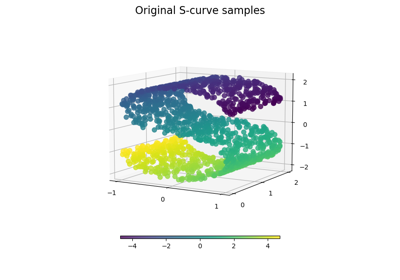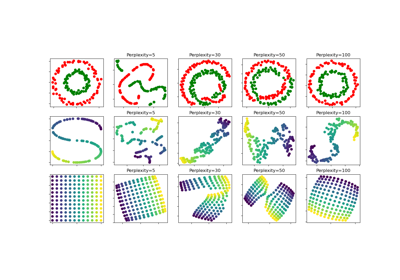# sklearn.datasets.make_s_curve¶

sklearn.datasets.make_s_curve(n_samples=100, *, noise=0.0, random_state=None)[source]

Generate an S curve dataset.

Read more in the User Guide.

Parameters
n_samplesint, default=100

The number of sample points on the S curve.

noisefloat, default=0.0

The standard deviation of the gaussian noise.

random_stateint or RandomState instance, default=None

Determines random number generation for dataset creation. Pass an int for reproducible output across multiple function calls. See Glossary.

Returns
Xarray of shape [n_samples, 3]

The points.

tarray of shape [n_samples]

The univariate position of the sample according to the main dimension of the points in the manifold.

## Examples using sklearn.datasets.make_s_curve¶